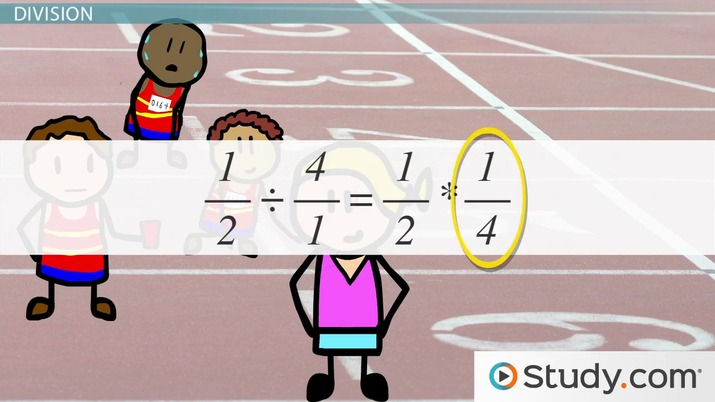Using Fraction Notation: Addition, Subtraction, Multiplication & Division

Lesson Transcript
Instructor: Jeff Calareso

Jeff teaches high school English, math and other subjects. He has a master's degree in writing and literature.

In mathematics, a fraction is a number that is not whole, and mathematical equations that contain fractions can be challenging to understand and solve. Learn how to use fraction notations to make it easier to perform addition, subtraction, multiplication, and division in problems with fractions. Updated: 10/02/2021

Fraction Notation

The term fraction notation just means a fraction written as a/b. We call the number above the line the numerator. The one below the line is the denominator.

If it rains five days in a week, well, that's a dreary week. In fraction notation, we'd say it rained 5/7 days. The denominator represents the total number of days in the week. The numerator is the part of the whole, or the number of days it rained.

What if we're in a Beatles song, and it rains eight days a week? Our fraction would be 8/7. That's called an improper fraction. It also breaks the calendar. But it's still a fraction written in fraction notation.

In this lesson, we're going to learn how to do all the fun things you might want to do with fractions: addition, subtraction, multiplication and division. Whoa. That's a lot. But don't worry. We'll start simple and build from there.An error occurred trying to load this video.

Try refreshing the page, or contact customer support.

Coming up next: Factoring Out Variables: Instructions & Examples

You're on a roll. Keep up the good work!

Replay
Your next lesson will play in 10 seconds
• 0:02 Fraction Notation
• 0:59 Multiplication
• 1:57 Division
• 4:55 Subtraction
• 5:42 Lesson Summary
Save Save

Want to watch this again later?

Timeline
Autoplay
Autoplay
Speed Speed

Multiplication

You might think we'd start with addition, which is so often the simplest operation. But with fraction notation, multiplication is actually the easiest.

When we multiply fractions, a/b * c/d = ac/bd. In other words, 2/3 * 5/7 equals 2 * 5 over 3 * 7. That's 10/21.

Let's see that in action. Let's say there's 1/2 of a pie just sitting on the kitchen counter, begging to be eaten. You decide to eat 1/3 of what's there. That 1/2 * 1/3. We just multiply the numerators, 1 * 1, to get 1. Then we multiply the denominators, 2 * 3, to get 6. How much of the pie did you eat? 1/6. As you can see, there were originally 6 pieces, so your 1/3 of 1/2 is 1/6 of the original pie.

Division

Let's tackle division next. When we divide fractions, (a/b) / (c/d) = a/b * d/c. Wait, what? When we divide fractions, we take the reciprocal of the second fraction, and then multiply them together. In other words, flip the second fraction upside down, then multiply.

So, 2/3 divided by 5/7 equals 2/3 * 7/5. That's 14/15.

Should we see it in action? Ok. Let's say you're working off that pie by running a half marathon. But you only had a little pie, so you're running as part of a 4-person relay team. What fraction of a marathon are you running? That's 1/2, or half the marathon, divided by 4 people, or 4/1.

To figure out (1/2) / (4/1), we take the reciprocal of 4/1. Again, just flip it upside down, like how your stomach feels if you go running too soon after eating pie. So 4/1 becomes 1/4. Then multiply 1/2 * 1/4. That's 1/8. So you'll run 1/8 of a full marathon. That's not bad!

Ok, time to talk addition. When we add fractions, we find a common denominator. Then add the numerators. We can't add 1/2 and 1/4, but we can add 2/4 and 1/4, which is 3/4.

Let's think about what this means. Let's say you have a box of 12 doughnuts. You eat one, or 1/12, of the doughnuts. Your friend eats 1/3 of the doughnuts. How do you compare 1/12 and 1/3? It's like your friend is trying to hide how many doughnuts he ate. Not cool.

To unlock this lesson you must be a Study.com Member.

Register to view this lesson

Are you a student or a teacher?